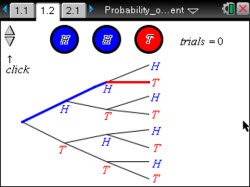# Activities

••• ##### Subject Area

• Math: Algebra II: Probability

• ##### Author9-12

45 Minutes

• ##### Device
•TI-Nspire™ CX/CX II
•TI-Nspire™ CX CAS/CX II CAS
• TI-Nspire™ Navigator™
• TI-Nspire™
• TI-Nspire™ CAS
• ##### Software

TI-Nspire™
TI-Nspire™ CAS

3.0

• ##### Report an Issue

Probability of Repeated Independent Events#### Activity Overview

Investigate probability by simulating tossing a coin three times.

#### Objectives

• Students will identify the sample space of an experiment involving repeated independent trials.
• Students will calculate the probability of multiple independent events occurring.
• Students will gain understanding of the distinction of the relationship between theoretical and experimental probability.
• Reason abstractly and quantitatively. (CCSS Mathematical Practice)
• Look for and express regularity in repeated reasoning. (CCSS Mathematical Practice)

#### Vocabulary

• experiment
• outcomes
• sample space
• event
• independent event
• dependent event
• probability tree diagram

#### About the Lesson

This lesson involves an investigation of probability by simulating tossing a coin three times.

As a result, students will:

• Understand probability of specific outcomes of repeated independent trials.
• Understand using a probability tree diagram to determine probability.